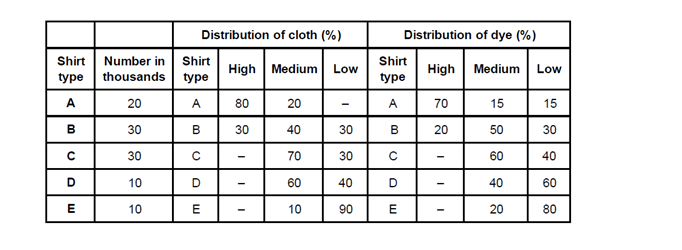Answer the questions based on the following information.
A clothing company produces five types of shirts — A, B, C, D and E — using cloth of three qualities — high, medium and low and using dyes of three qualities — high, medium and low. One shirt requires 1.5 m of cloth. The following table gives respectively:
1. The number of shirts (of each category) produced, in thousands
2. The percentage distribution of cloth quality in each type of shirt, and
3. The percentage distribution of dye quality in each type of shirtQuestion 1: What is the total requirement of cloth?
a. 1,50,000 m
b. 2,00,000 m
c. 2,25,000 m
d. 2,50,000 m

Question 2: How many metres of high-quality cloth is consumed?
a. 22,500 m
b. 37,500 m
c. 46,500 m
d. 40,000 m

Question 3: How many metres of medium quality dyeis consumed by B-type shirts?
a. 8,000 m
b. 112,000 m
c. 22,500 m
d. 30,500 m

Question 4: What is the ratio of the three qualities of dyes in high-quality cloth?
a. 2 : 3 : 5
b. 1 : 2 : 5
c. 7 : 9 : 10
d. Cannot be determined

Question 5: What is the ratio of low-quality dye used for D-type shirts to that used for E- type shirts?
a. 3 : 2
b. 3 : 4
c. 1 : 2
d. 2 : 3

We can calculate total number of shirts by obtaining the quantities form the table

Given, Cloth required per shirt = 1.5m

Total requirement of cloth= Total number of shirts(in thousands) × Cloth required per shirt

= (20 + 30 + 30 + 10 + 10) 1000 × 1.5 = 1,50,000 m.

Cloth required per shirt = 1.5m

Total high quality cloth consumed

= 1.5 (80% of 20000 + 30% of 30000)= 37,500 m.

From the table we know that 50% of medium- quality dye was used for B-type shirts.

Total quantity of medium quality dye consumed by B-type shirts = (50% of 30000) × 1.5 = 22,500 m.

From the given data in the table we can only know the relationship between the type of shirt and amount of cloth used and type of shirt and dye used. We cannot determine the ratio of a certain quality of dyes for certain cloth quality as the data is insufficient. Hence, the answer is cannot be determined.